Скачать презентацию SCATTERED DATA VISUALIZATION Yingcai Xiao Scattered Data

• Количество слайдов: 37SCATTERED DATA VISUALIZATION Yingcai Xiao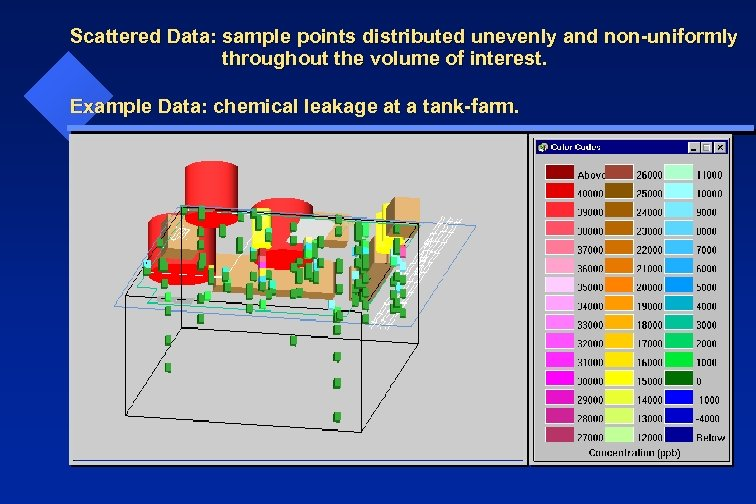Scattered Data: sample points distributed unevenly and non-uniformly throughout the volume of interest. Example Data: chemical leakage at a tank-farm.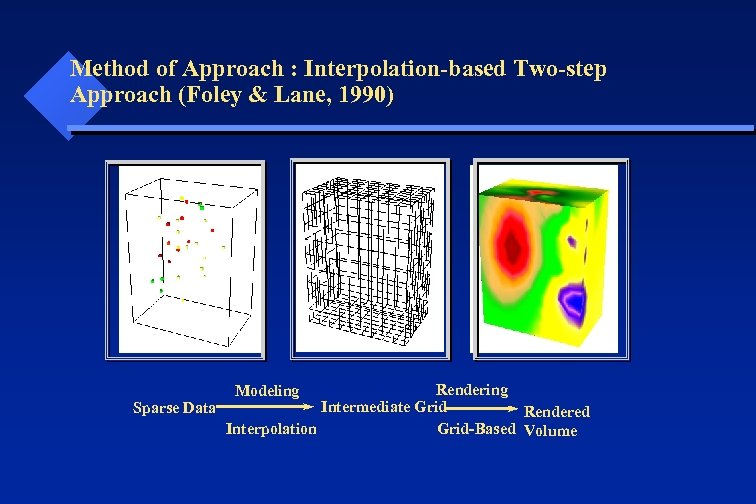Method of Approach : Interpolation-based Two-step Approach (Foley & Lane, 1990) Rendering Intermediate Grid Sparse Data Rendered Interpolation Grid-Based Volume Modeling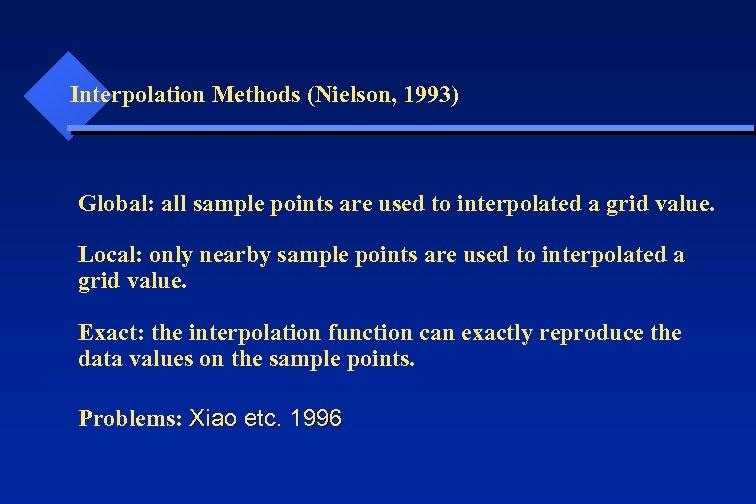Interpolation Methods (Nielson, 1993) Global: all sample points are used to interpolated a grid value. Local: only nearby sample points are used to interpolated a grid value. Exact: the interpolation function can exactly reproduce the data values on the sample points. Problems: Xiao etc. 1996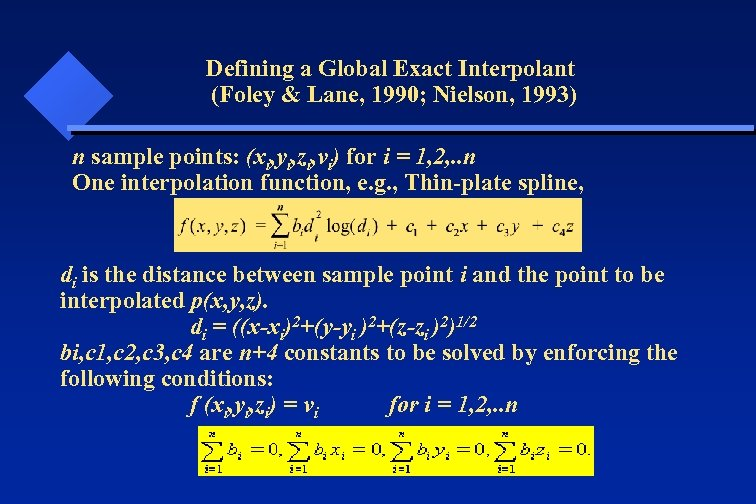Defining a Global Exact Interpolant (Foley & Lane, 1990; Nielson, 1993) n sample points: (xi, yi, zi, vi) for i = 1, 2, . . n One interpolation function, e. g. , Thin-plate spline, di is the distance between sample point i and the point to be interpolated p(x, y, z). di = ((x-xi)2+(y-yi )2+(z-zi )2)1/2 bi, c 1, c 2, c 3, c 4 are n+4 constants to be solved by enforcing the following conditions: f (xi, yi, zi) = vi for i = 1, 2, . . n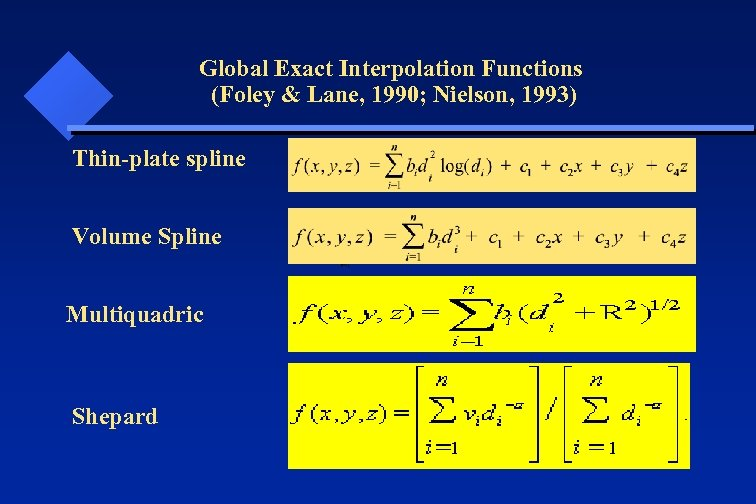Global Exact Interpolation Functions (Foley & Lane, 1990; Nielson, 1993) Thin-plate spline Volume Spline Multiquadric Shepard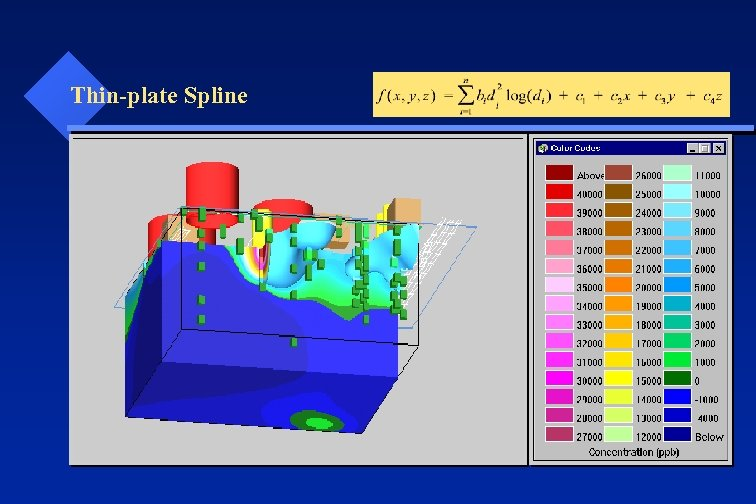Thin-plate Spline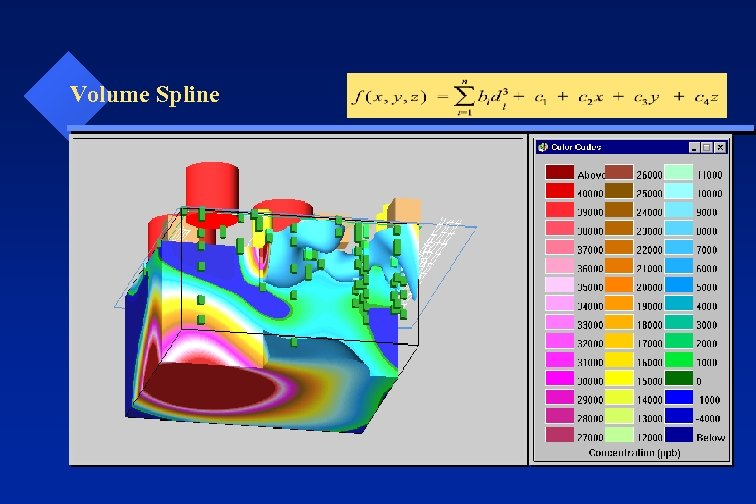Volume Spline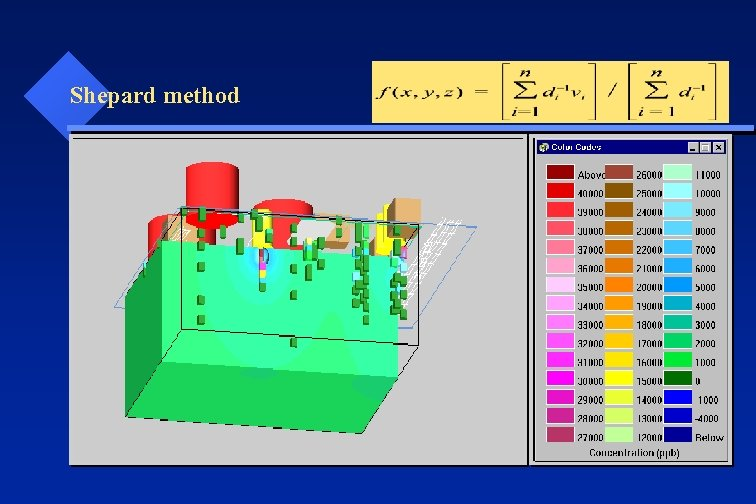Shepard method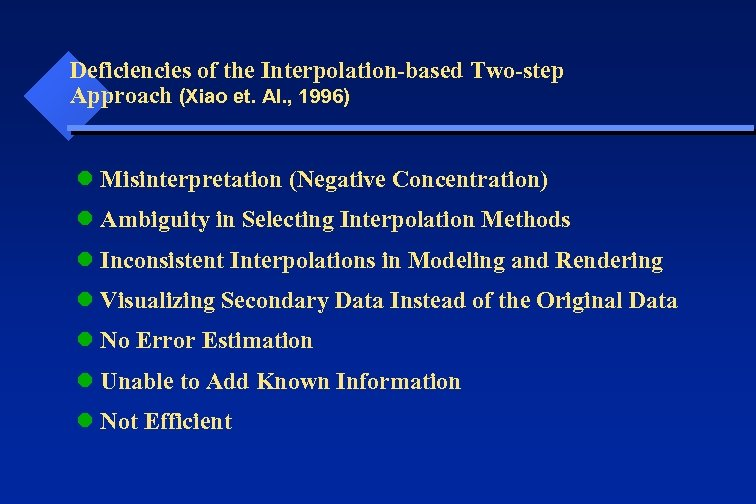Deficiencies of the Interpolation-based Two-step Approach (Xiao et. Al. , 1996) l Misinterpretation (Negative Concentration) l Ambiguity in Selecting Interpolation Methods l Inconsistent Interpolations in Modeling and Rendering l Visualizing Secondary Data Instead of the Original Data l No Error Estimation l Unable to Add Known Information l Not Efficient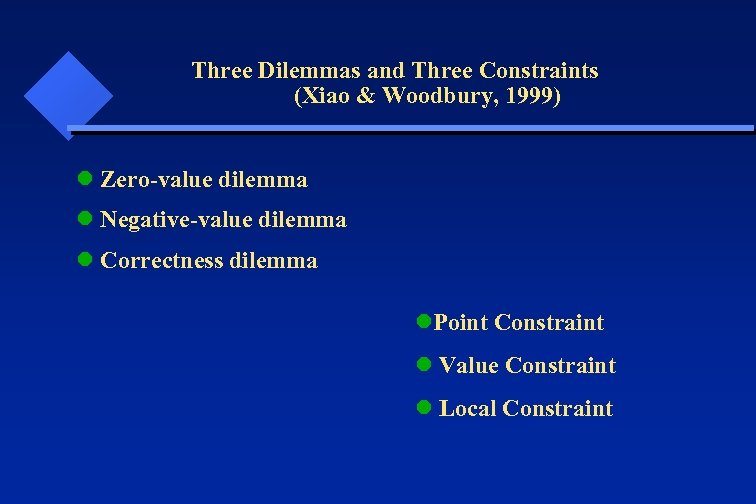Three Dilemmas and Three Constraints (Xiao & Woodbury, 1999) l Zero-value dilemma l Negative-value dilemma l Correctness dilemma l. Point Constraint l Value Constraint l Local Constraint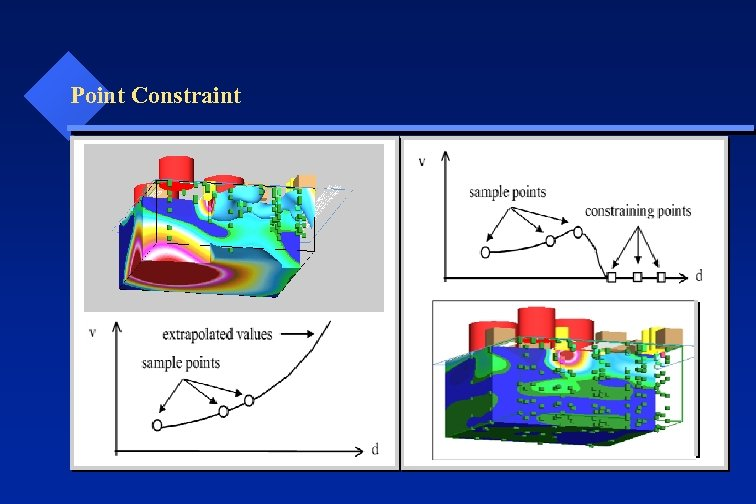Point Constraint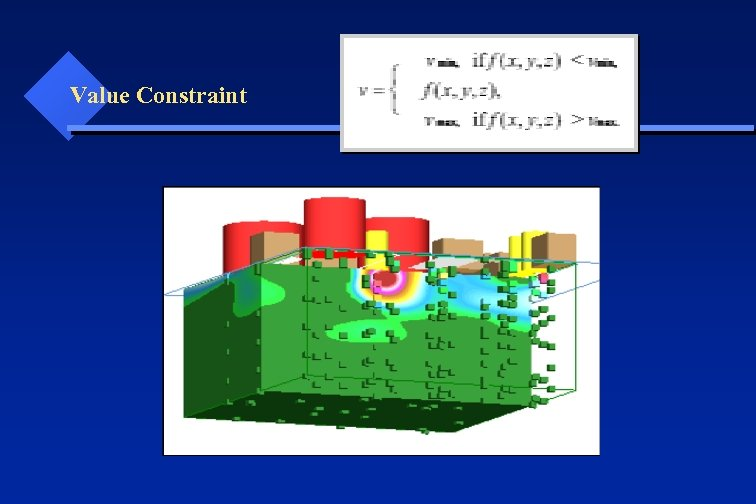Value Constraint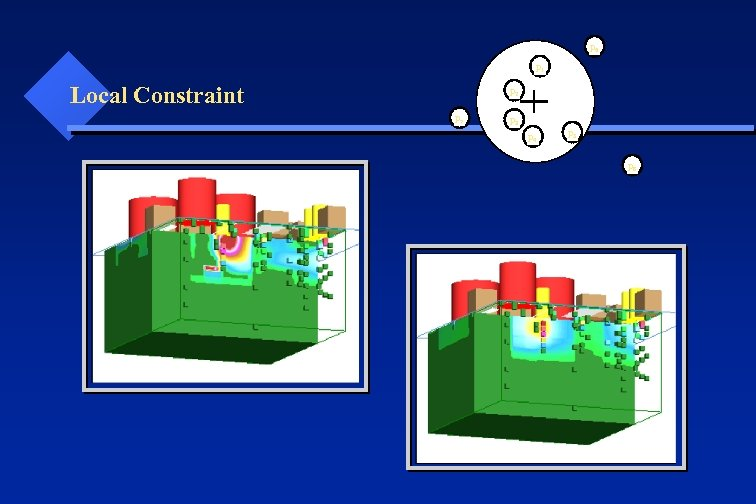p 6 p 1 Local Constraint p 2 p 7 p 3 p 4 p 5 p 8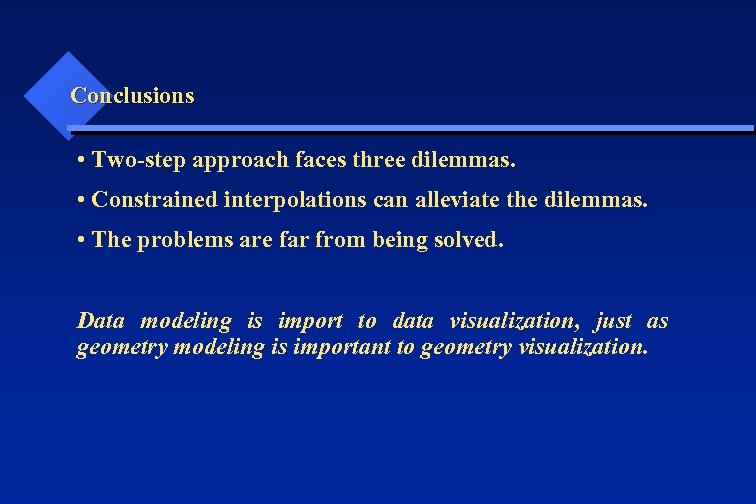Conclusions • Two-step approach faces three dilemmas. • Constrained interpolations can alleviate the dilemmas. • The problems are far from being solved. Data modeling is import to data visualization, just as geometry modeling is important to geometry visualization.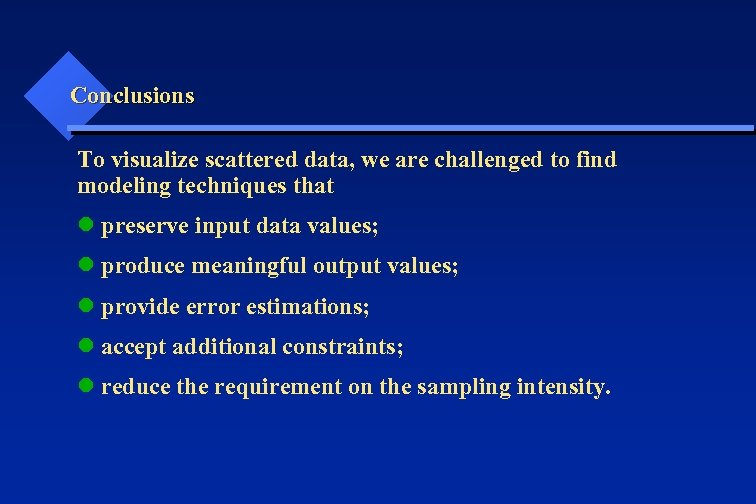Conclusions To visualize scattered data, we are challenged to find modeling techniques that l preserve input data values; l produce meaningful output values; l provide error estimations; l accept additional constraints; l reduce the requirement on the sampling intensity.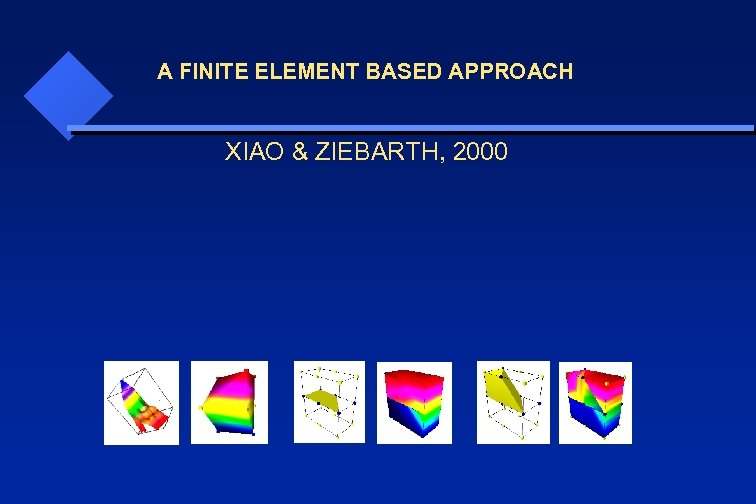A FINITE ELEMENT BASED APPROACH XIAO & ZIEBARTH, 2000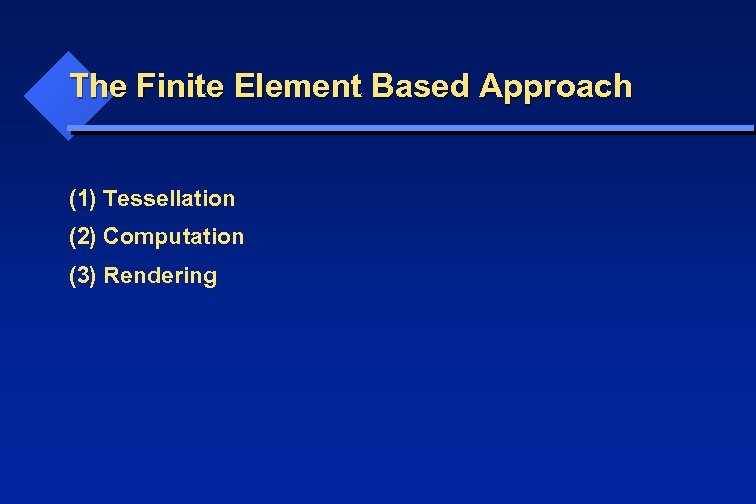The Finite Element Based Approach (1) Tessellation (2) Computation (3) Rendering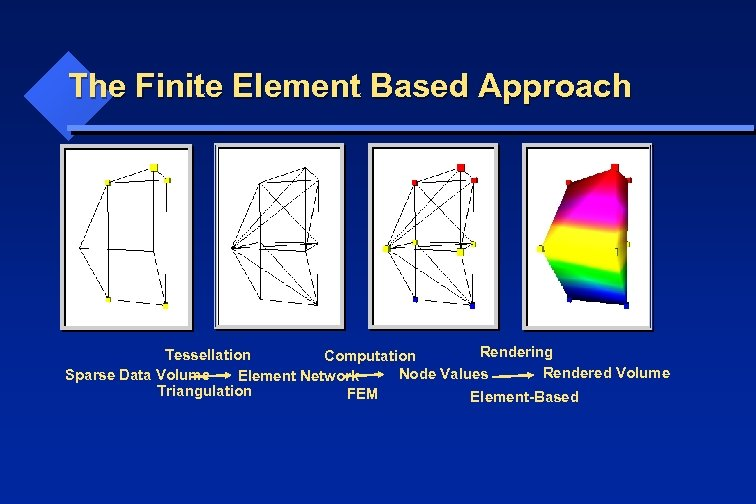The Finite Element Based Approach Rendering Tessellation Computation Rendered Volume Node Values Sparse Data Volume Element Network Triangulation FEM Element-Based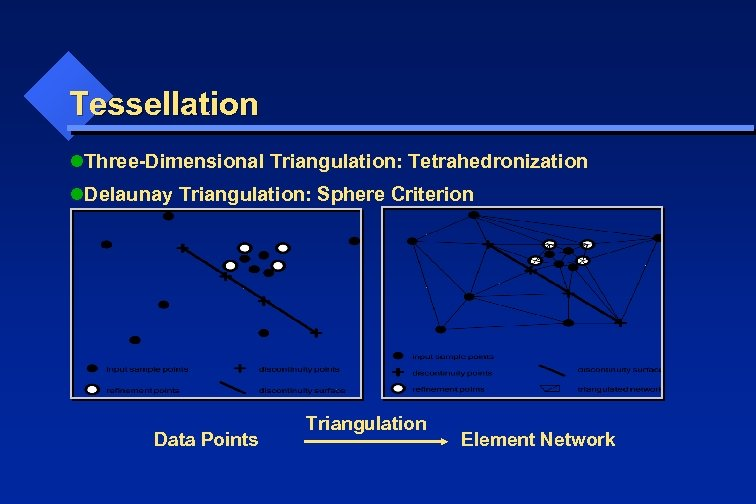Tessellation l. Three-Dimensional Triangulation: Tetrahedronization l. Delaunay Triangulation: Sphere Criterion Data Points Triangulation Element Network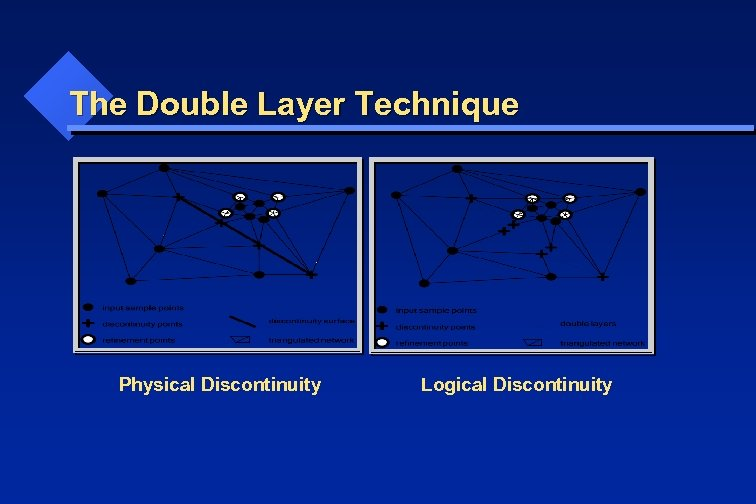The Double Layer Technique Physical Discontinuity Logical Discontinuity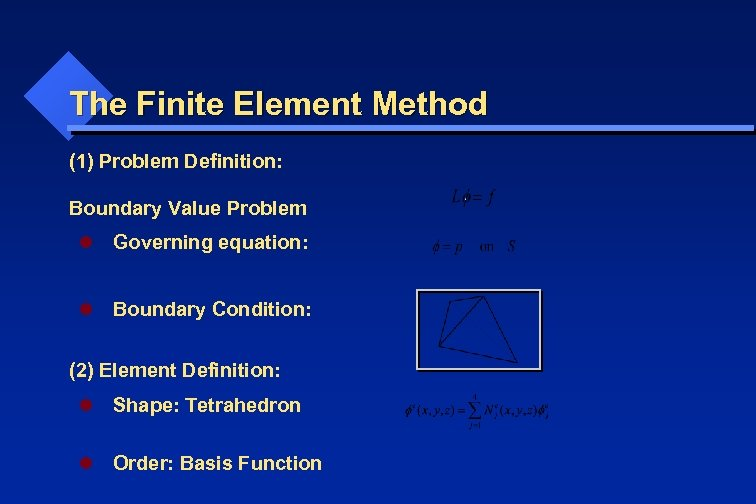The Finite Element Method (1) Problem Definition: Boundary Value Problem l Governing equation: l Boundary Condition: (2) Element Definition: l Shape: Tetrahedron l Order: Basis Function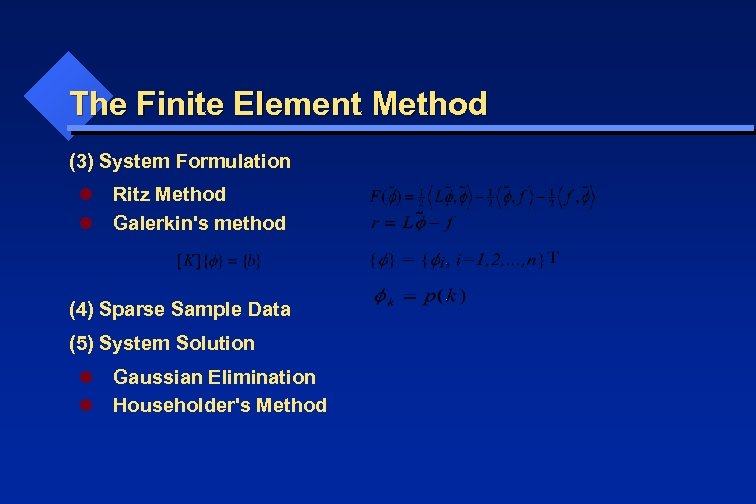The Finite Element Method (3) System Formulation l Ritz Method l Galerkin's method (4) Sparse Sample Data (5) System Solution l Gaussian Elimination l Householder's Method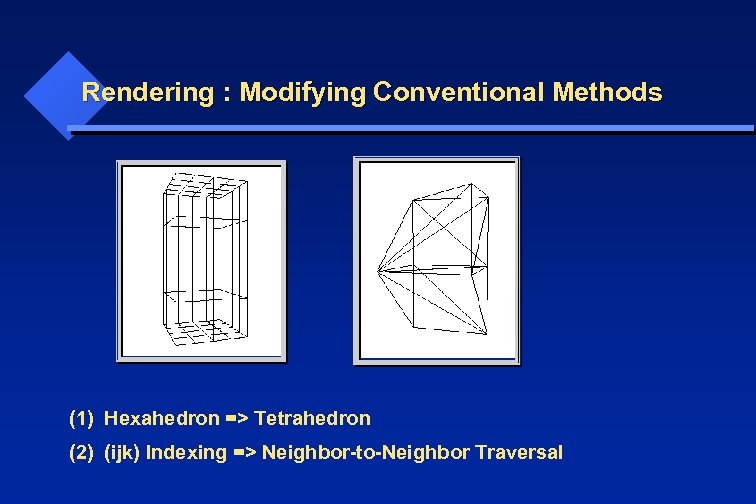Rendering : Modifying Conventional Methods (1) Hexahedron => Tetrahedron (2) (ijk) Indexing => Neighbor-to-Neighbor Traversal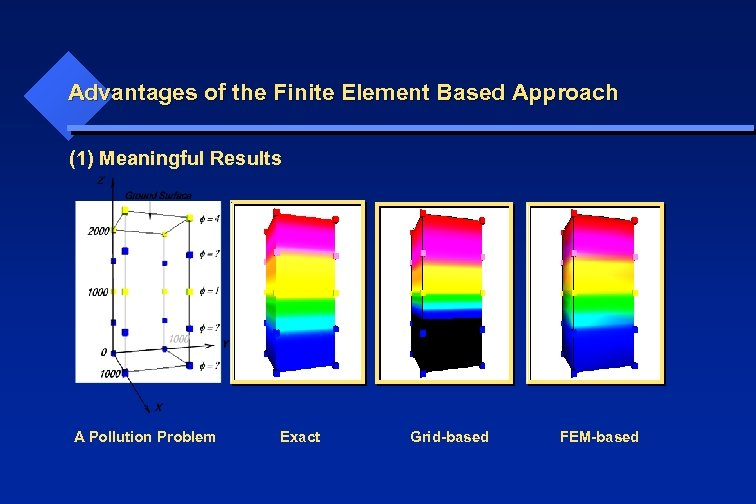Advantages of the Finite Element Based Approach (1) Meaningful Results A Pollution Problem Exact Grid-based FEM-based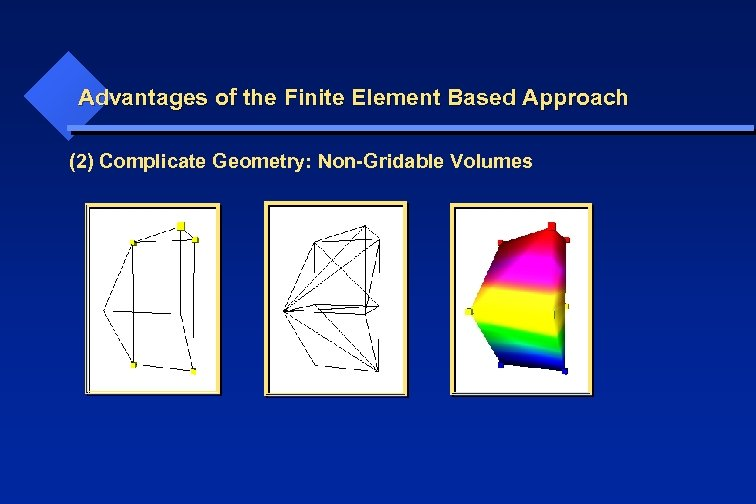Advantages of the Finite Element Based Approach (2) Complicate Geometry: Non-Gridable Volumes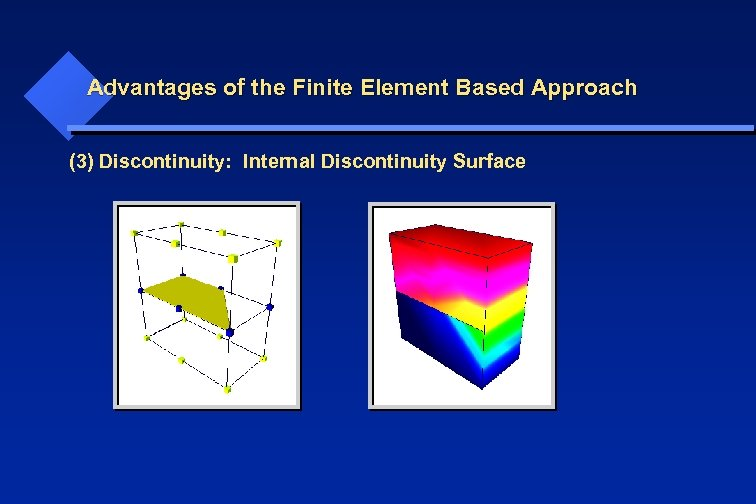Advantages of the Finite Element Based Approach (3) Discontinuity: Internal Discontinuity Surface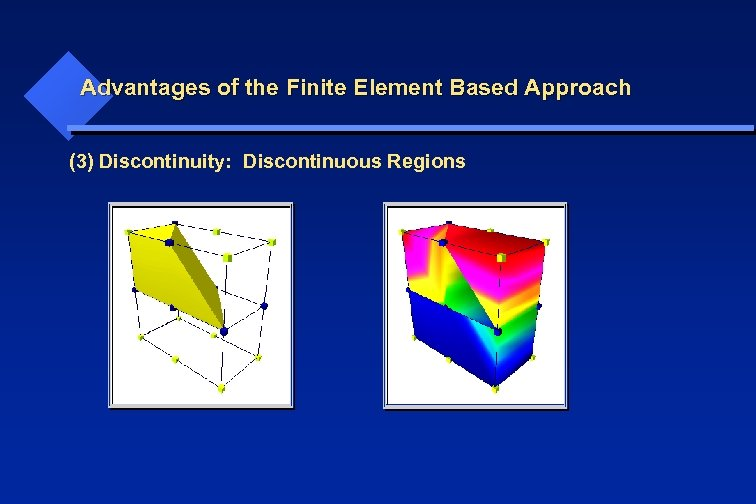Advantages of the Finite Element Based Approach (3) Discontinuity: Discontinuous Regions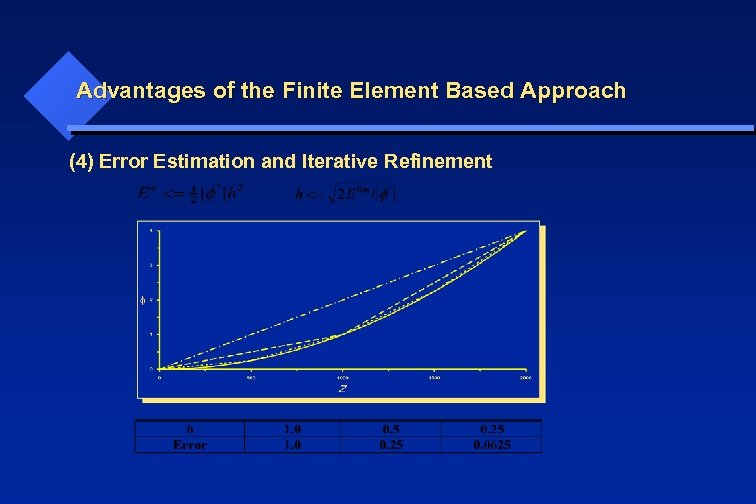Advantages of the Finite Element Based Approach (4) Error Estimation and Iterative Refinement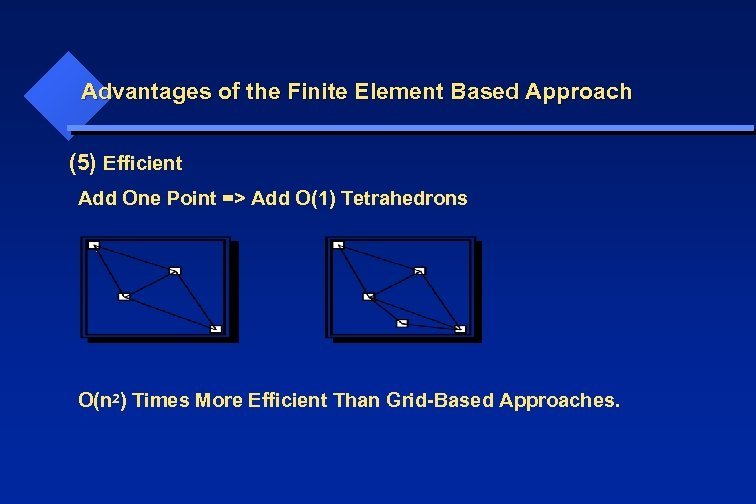Advantages of the Finite Element Based Approach (5) Efficient Add One Point => Add O(1) Tetrahedrons O(n 2) Times More Efficient Than Grid-Based Approaches.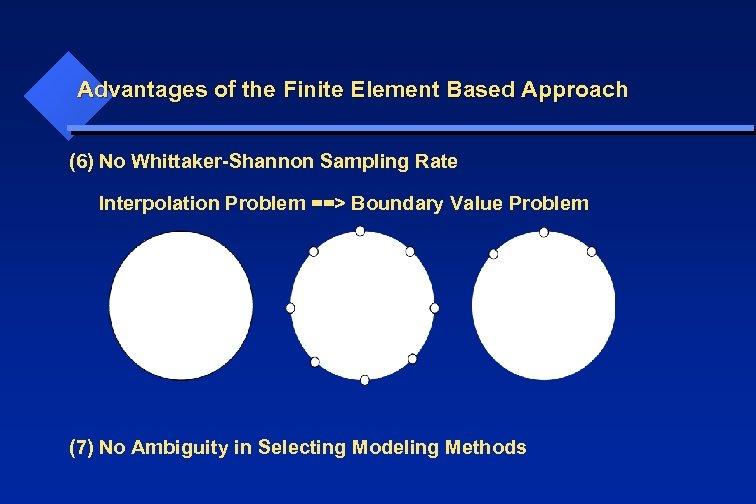Advantages of the Finite Element Based Approach (6) No Whittaker-Shannon Sampling Rate Interpolation Problem ==> Boundary Value Problem (7) No Ambiguity in Selecting Modeling Methods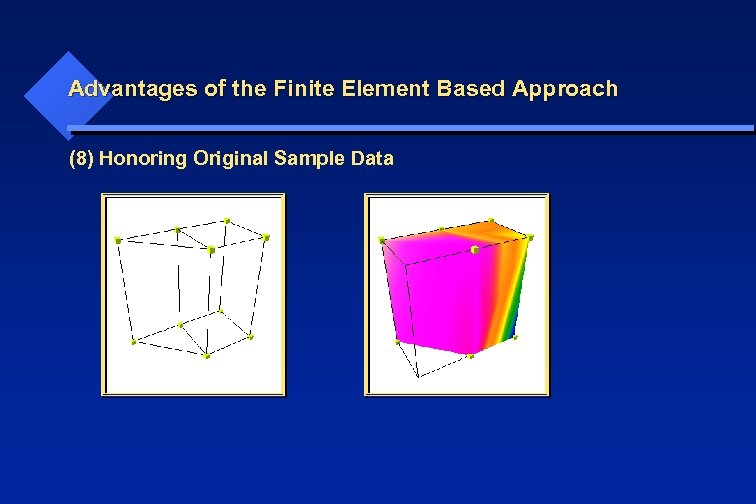Advantages of the Finite Element Based Approach (8) Honoring Original Sample Data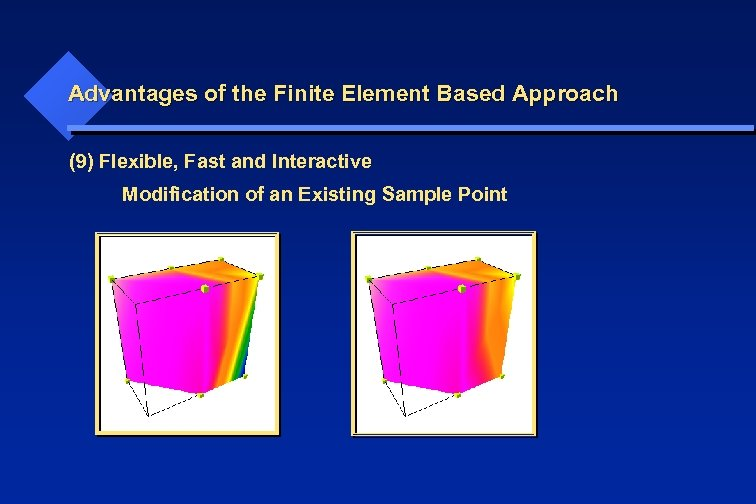Advantages of the Finite Element Based Approach (9) Flexible, Fast and Interactive Modification of an Existing Sample Point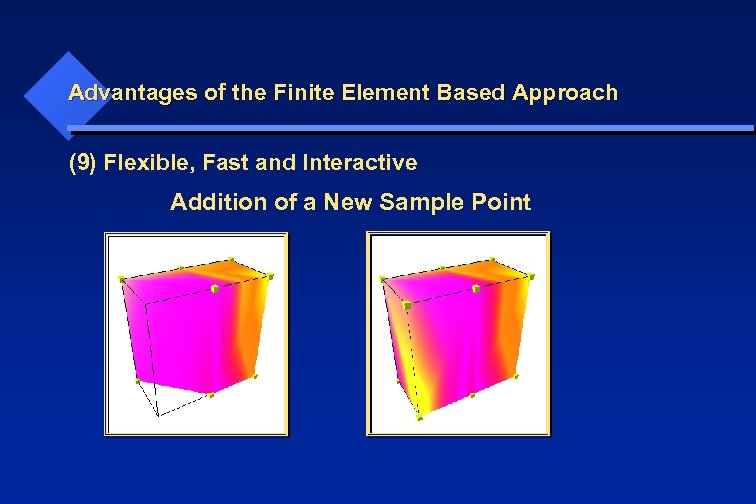Advantages of the Finite Element Based Approach (9) Flexible, Fast and Interactive Addition of a New Sample Point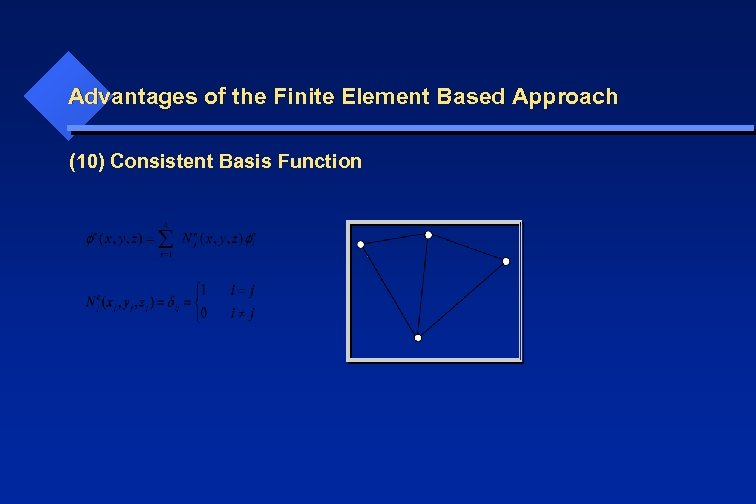Advantages of the Finite Element Based Approach (10) Consistent Basis Function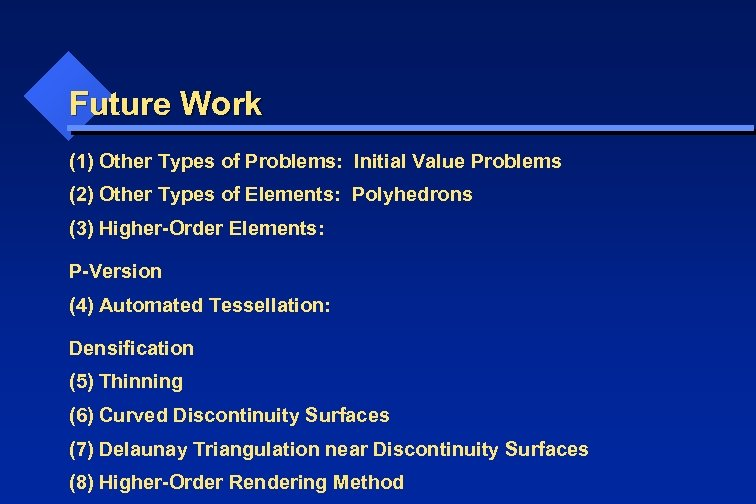Future Work (1) Other Types of Problems: Initial Value Problems (2) Other Types of Elements: Polyhedrons (3) Higher-Order Elements: P-Version (4) Automated Tessellation: Densification (5) Thinning (6) Curved Discontinuity Surfaces (7) Delaunay Triangulation near Discontinuity Surfaces (8) Higher-Order Rendering Method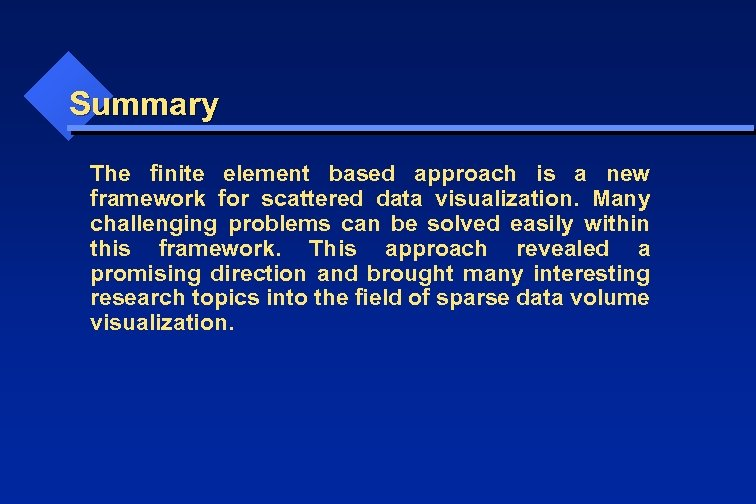Summary The finite element based approach is a new framework for scattered data visualization. Many challenging problems can be solved easily within this framework. This approach revealed a promising direction and brought many interesting research topics into the field of sparse data volume visualization.